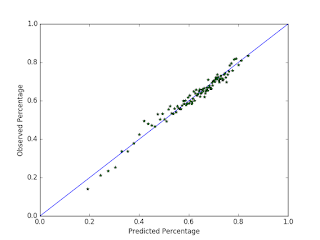## Posts

Showing posts from May, 2016

### Beat the Streak: Day FiveWith the recent high offensive production in the mlb, many people have amassed large streaks in the Beat the Streak contest.  The current leader has a streak of 41 games, which he got by picking exclusively red sox players.  Many other people have streaks in the high 30s, and I have a streak of 19 myself right now.  It seems like a lot more people have been getting longer streaks this year.  Some of this is probably due to the fact that more batters are getting hits this year than they have in the past, but it is probably also due to MLB.com's new pick selection system, which makes it easier than ever to make high quality picks using whatever strategy you want.  I would not be surprised if this is the year somebody wins.  If that's the case, this could be one of my last blog posts on this topic.     In this blog post, I am going to evaluate my current pick selection strategy  by testing it on data from 2015.  My data consists of a list of observations, where each observation c

### Improving Naive Bayes with MLE

Today I'm going to be talking about the probabilistic classifier known as Naive Bayes, and a recent idea I came up with to improve it. My idea relies on the same assumptions that naive bayes does, but it finds different values for the conditional probabilities and class probabilities that describe the data better (or so I originally thought). In this blog post, I am going to quickly go through the traditional naive bayes setup, introduce my idea, then compare the two in terms of prediction quality. The Traditional Setup Let's assume we have a data set with $N$ observations, where each observation has $n$ attributes $x_1, x_2, \dots, x_n$ and $1$ class value $C_k$. Naive bayes says that we can compute the probability of observing $C_k$ given the attribute information by evaluating the following formula: $$P(C_k | x_1, \dots, x_n) = \frac{ P(C_k) \prod_{i=1}^n P(x_i \mid C_k) }{P(x_1, \dots, x_n)}$$ where  P(x_1